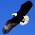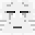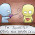Wednesday, 30 January 2013

20130130 Point to Ponder... Rational Numbers on a Number Line?

Enter your response to the following in "Comments".

1.No, I do not. ,-5,-4,-3,-2,-1,1,2,3,4,5 are all rational numbers and they. fit the requirements of the question, henceforth the question is valid

2.I do not agree as we can represent -5 on the number line example , -5,-4,-3,-2,-1,0,1,2,3,4,5.So the question is valid.

3.I do not agree.-5 is part of real numbers.Basically real numbers are any number(Decimal,rational,irrational,whole,etc.)except imaginary numbers and infinity.-5 is a rational number.rational numbers are part of the real numbers.5 is a rational as it is 5/1.So -5 is almost the same but it is expressed as -5/1

1.This comment has been removed by the author.

2.My opinion has changed.Actually i kinda agree with Juliet.Rational numbers such as 5.2 can be stated but the problem is if i say that the rational numbers are from -5 to 4 its actually wrong.Because 3.7495746 is not a rational number so we can never state rational numbers in a number line.

4.No, as we can represent 1which is a rational number on a number line :-5,-4,-3,-2,-1,0,1,2,3,4,5

5.No, I do not agree. -5,-4,-3,-2,-1,1,2,3,4,5 are rational numbers and they meet the requirements of the question, so the question is valid

6.I do not agree with Juliet. rational numbers can be written in the number line. the rules for m is that m has to be a rational number that is both larger or equals to -5 and smaller than 4 therefore it is still possible to write the statement in a number line and thus making the question valid

7.I do not agree as the numbers between -5 and 4 are all rational numbers and they can be used to answer the question. Thus,the question is valid.

8.No I do NOT AGREE with Juliet. It is possible to express the requirements of the question on a number line.
-5,-4,-3,-2,-1,0,1,2,3

9.I don't agree with Juliet because rational numbers like 3,2,1,0,-1,-2,-3,-4,-5 can all be written on the number line and thus the question is valid.

10.No, I do not agree with Juliet, because rational numbers eg. -4, -3, -2, -1, 0, 1, 2, 3, 4. can be written on the number line and therefore, the question is valid.

11.we can put rational numbers on the number line. thus, julian is not correct

12.No. I do not agree because rational numbers like -3 -2 -1 0 1 2 3, can be written on the number line. And thus the question is valid.

13.I don't agree because rational numbers like -5 -4 -3 -2 -1 0 1 2 3 4, can be written on the number line. Hence, the question should be valid.

14.I do not agree with Juliet because -5 -4 -3 -2 -1 0 1 2 3 4 are rational and can be written on the number line. So the question is valid.

15.No, i do not agree as -5 -4 -3 -2 -1 0 1 2 3 4 are all rational numbers so the question should be vaild

16.I do not agree with Juliet as rational numbers such as -4, -3, -2, -1, 0, 1, 2, 3, 4 are all rational numbers and they fit on a number line.

17.I do not agree with Juliet because -5, -4, -3, -2, -1, 0, 1, 2, 3 and 4 are rational numbers and they also can be written on a number line. I conclude that the question is valid

18.Actually i kinda agree with Juliet.Rational numbers such as 5.2 can be stated but the problem is if i say that the rational numbers are from -5 to 4 its actually wrong.Because 3.7495746 is not a rational number so we can never state rational numbers in a number line.